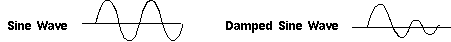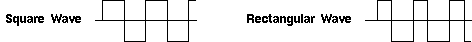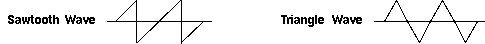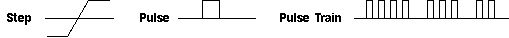Home > Electronic Tutorials > Oscilloscope Tutorial > Oscilloscope Types of Waves

Online Oscilloscope Tutorial

# About OSCILLOSCOPE Oscilloscope Types of Waves

## Types of Waves in Oscilloscope

You can classify most waves into these types:

• Sine waves
• Square and rectangular waves
• Triangle and sawtooth waves
• Step and pulse shapes

### Sine Waves

The sine wave is the fundamental wave shape for several reasons. It has harmonious mathematical properties - it is the same sine shape you may have studied in high school trigonometry class. The voltage in your wall outlet varies as a sine wave. Test signals produced by the oscillator circuit of a signal generator are often sine waves. Most AC power sources produce sine waves. (AC stands for alternating current, although the voltage alternates too. DC stands for direct current, which means a steady current and voltage, such as a battery produces.)

The damped sine wave is a special case you may see in a circuit that oscillates but winds down over time.

Following Figure shows examples of sine and damped sine waves.Sine and Damped Sine Waves

### Square and Rectangular Waves

The square wave is another common wave shape. Basically, a square wave is a voltage that turns on and off (or goes high and low) at regular intervals. It is a standard wave for testing amplifiers - good amplifiers increase the amplitude of a square wave with minimum distortion. Television, radio, and computer circuitry often use square waves for timing signals.

The rectangular wave is like the square wave except that the high and low time intervals are not of equal length. It is particularly important when analyzing digital circuitry.

Following Figure shows examples of square and rectangular waves.Square and Rectangular Waves

### Sawtooth and Triangle Waves

Sawtooth and Triangle waves result from circuits designed to control voltages linearly, such as the horizontal sweep of an analog oscilloscope or the raster scan of a television. The transitions between voltage levels of these waves change at a constant rate. These transitions are called ramps.

Following Figure shows examples of sawtooth and triangle waves.Sawtooth and Triangle Waves

### Step and Pulse Shapes

Signals such as steps and pulses that only occur once are called single-shot or transient signals. The step indicates a sudden change in voltage, like what you would see if you turned on a power switch. The pulse indicates what you would see if you turned a power switch on and then off again. It might represent one bit of information traveling through a computer circuit or it might be a glitch (a defect) in a circuit.

A collection of pulses traveling together creates a pulse train. Digital components in a computer communicate with each other using pulses. Pulses are also common in x-ray and communications equipment.

Figure 6 shows examples of step and pulse shapes and a pulse train.Step, Pulse, and Pulse Train Shapes